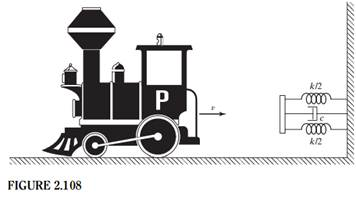+1-415-670-9189
info@expertsmind.com

# Get Solution

Determine the time taken to reach the maximum displacement
Course:- Mechanical Engineering
Reference No.:- EM131146338

 TweetExpertsmind Rated 4.9 / 5 based on 47215 reviews.
Review Site
Assignment Help >> Mechanical Engineering

A railroad car of mass 2,000 kg traveling at a velocity v = 10 m/s is stopped at the end of the tracks by a spring-damper system, as shown in Fig. 2.108. If the stiffness of the spring is k = 80 N/mm and the damping constant is c = 20 N-s/mm determine (a) the maximum displacement of the car after engaging the springs and damper and (b) the time taken to reach the maximum displacement.Minimize

Ask Question & Get Answers from Experts
Browse some more (Mechanical Engineering) Materials
 Find the tension in the cable when the sub is (a) hanging above the water, and (b) once the sub is lowered into the sea water. When released from the cable, will the bell te A voltage signal consists of a pure sine wave of amplitude 10.0 V at 180 Hz. This signal is sampled digitally at a sampling frequency of 200 Hz. 128 data points are taken. Air contained in a rigid, insulated tank fitted with a paddle wheel, initially at 4 bar, 40oC and volume of 0.2 m^3, is stirred until its temperature is 500 K. Assuming the id Given that at typical liquid densities p σ3 ≈ 1 (p = N/V, and σ is the diameter of a molecule), estimate the mean free path of and collision frequency of a molecule in a roo The drag coefficient (Cd) of a semi-tractor trailer without a cab fairing is 0.96,and with the fairing is 0.76 based on frontal area of 80ft^2. if power=Force * velocity, dete A colleague indicates that this is improper because the measuring instrument is not in the far field of the antenna. Is the instrument in the near or the far field of the tr Hot combustion gases of a furnace are separated from the ambient air and its surroundings, which are at 25 C, by a brick wall that is 0.15m thick. The brick has a thermal cond A 500-N concrete block is to be lifted by the pair of tongs shown. Determine the smallest allowable value of the coefficient of static friction between the block and the ton